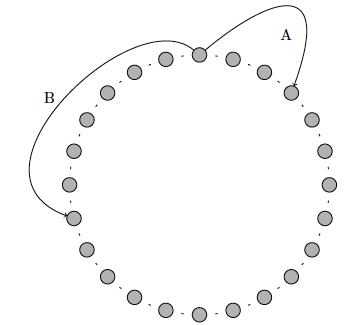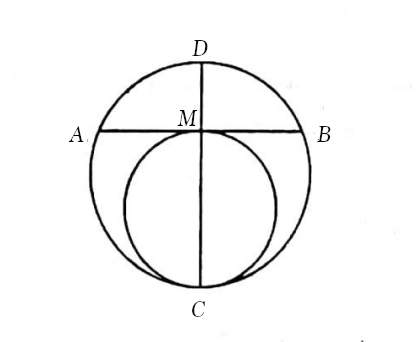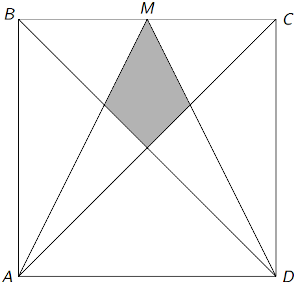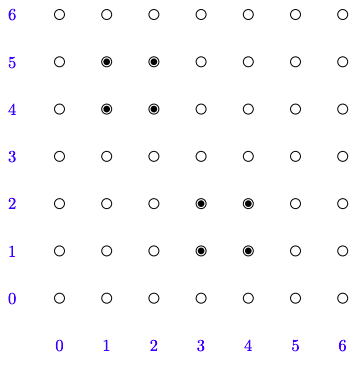MT #3: Full-syllabus

Timings: 10:30-13:30 Hrs    Date: 28 Feb 2021

Submission file: Write answers to all the ten questions on a single sheet of paper. Email a picture of your answer sheet. Name the file as PartA.jpg.

For this part, answers must be written without any explanation.

1. There are 8 marbles of different colors and 8 different bags of matching colors. In how many ways can we put one marble in each bag such that exactly 5 marbles go to the bag of the matching color?

2. Suppose $$f(x)$$ is a continous function satisfying two relations:
(a) $$f(x)-f\left(\frac{x}{4}\right)=\frac{3x}{4}$$
(b) $$f(0) = 3$$
Find $$f(x)$$.

3. Let $$z_{1}, z_{2}, z_{3}$$ be complex numbers such that $\left|z_{1}\right|=\left|z_{2}\right|=\left|z_{3}\right|=1$ and $\frac{z_{1}^{2}}{z_{2} z_{3}}+\frac{z_{2}^{2}}{z_{1} z_{3}}+\frac{z_{3}^{2}}{z_{1} z_{2}}+1=0$ Find the value of $$\left|z_{1}^3+z_{2}^3+z_{3}^3\right|$$.

4. There are $$n$$ stones arranged in a circular pattern. At time $$t=0$$ s, two grasshoppers are resting on a common stone. Grasshopper A starts to hop in clockwise direction in intervals of 3 stones at each time step. Grasshopper B jumps every 7 stones at each time step in anti-clockwise manner. The figure below shows the first jump. What is the earliest time step when both the grasshoppers land on the same stone again? Find this number when $$n=300$$ and when $$n=301$$.5. Four six-faced dice are rolled simultaneously. What is the probability that the highest-valued die shows 4? Write the answer as a reduced fraction.

6. Find the equation of the plane in $$R^3$$ that passes through the following points: $$(2,0,0), (1,3,1)$$ and $$(5,1,1)$$.

7. $$ABC$$ is a triangle with $$\angle A = 30^{\circ}$$, $$\angle B = 60^{\circ}$$ and $$AB=10$$. Find the length of the shortest trisector of $$\angle C$$.

8. A non-zero polynomial $$p(x) = a_nx^n + a^{n-1}x^{n-1} + \ldots + a_0$$ is said to be a palindrome if $$a_i = a_{n-i}$$ for $$i=0,\ldots,n$$. It can be proved that a polynomial is a palindrome if and only if $$p(x) = x^n p( \frac{1}{x} )$$. Which of these statements are true?
1. If $$p(x)$$ and $$q(x)$$ are palindromes then $$p(x)+q(x)$$ is also a palindrome.
2. If $$p(x)$$ and $$q(x)$$ are palindromes then $$p(x)q(x)$$ is also a palindrome.
3. Suppose $$p(x)$$ is a palindrome that can be factored as $$r(x)s(x)$$ where both $$r(x)$$ and $$s(x)$$ are polynomials with integer coefficients. Then both $$r(x)$$ and $$s(x)$$ are palindromes.
4. Suppose $$p(x)$$ is a palindrome that can be factored as $$r(x)s(x)$$ where both $$r(x)$$ and $$s(x)$$ are polynomials with integer coefficients. Then exactly one of $$r(x)$$ or $$s(x)$$ is a palindrome.

9. The value of $${}^{50}C_0 {}^{50}C_{1} + {}^{50}C_1 {}^{50}C_{2} + \ldots + {}^{50}C_{49} {}^{50}C_{50}$$ is:
1. $${}^{100}C_{49}$$
2. $${}^{100}C_{50}$$
3. $${}^{100}C_{48}$$
4. $$({}^{50}C_{25})^2$$

10. Find all possible pairs of integers $$(m, n)$$ which satisfy $$m^{2}+2 m-35=2^{n}$$.

Part B: Subjective questions

Submission files: Each question in this part must be answered on a page of its own. Name the files as B1.jpg, B2.jpg, etc. In case you have multiple files for the same question, say B4, name the corresponding files as B4-1.jpg, B4-2.jpg, etc.

Clearly explain your entire reasoning. No credit will be given without reasoning. Partial solutions may get partial credit.

B1. We have two circles touching each other as shown below. The radius of the smaller circle is $$r$$. $$CM$$ is the diameter of the inner circle. $$AB$$ is a tangent to the inner circle with $$M$$ as its midpoint. Suppose $$|MA|=|MB|=a$$ cm. Find the radius of the larger circle in terms of $$a$$ and $$r$$.B2 (a) [4 marks] Find all positive integer solutions to the equation: $86x + 10y = 500$
You may find the following fact useful: $$(500,-4250)$$ is an integer solution to the above equation.

B2 (b) [6 marks] Let us suppose $$a,b,c$$ are positive integers. Suppose the equation $$ax+by = c$$ has $$m$$ positive solutions. How many positive solutions does the following equation have? $ax+by = c+ab$

B3. In the figure shown below, ABCD is a square with side length 1 unit. M is the midpoint of the side BC. What is the area of the shaded portion?B4 (a)$\int_{0}^{1} x(1-x)^{2021} \, dx$
B4 (b)$\int_{0}^{\pi / 2} \frac{d x}{1+(\tan x)^{2021}}$

B5. We are given a number $$P$$. We want to pick $$n$$ positive integers $$x_1,\ldots,x_n$$ such that two conditions are met:
Condition 1: The product of the $$x_i$$s must be $$P$$. That is, $$x_1\times x_2\times\ldots x_n = P$$.
Condition 2: The GCD of $$x_1,\ldots,x_n$$ is maximized.

(a) Let us denote the GCD by $$g$$. If the prime factorization of $$P=p_{1}^{k_{1}} \ldots p_{\ell}^{k_{\ell}}$$, what is the value of $$g$$?
(b) Write the value of $$g$$ for $$n=5$$ and $$P=2^5 \cdot 3^6\cdot 5^{10} \cdot 7^3$$.

B6. We have a square grid where $$(0,0)$$ is the origin and $$(6,6)$$ is the top-right corner. We want to move from the origin to $$(6,6)$$ using only right steps and upward steps. It is a well known fact that there are $${}^{12}C_6$$ ways of doing this. Consider the following addtional constraint: We are not allowed to step on the eight shaded coordinates shown in the figure below. How many ways are there to go from $$(0,0)$$ to $$(6,6)$$ obeying these constraints?